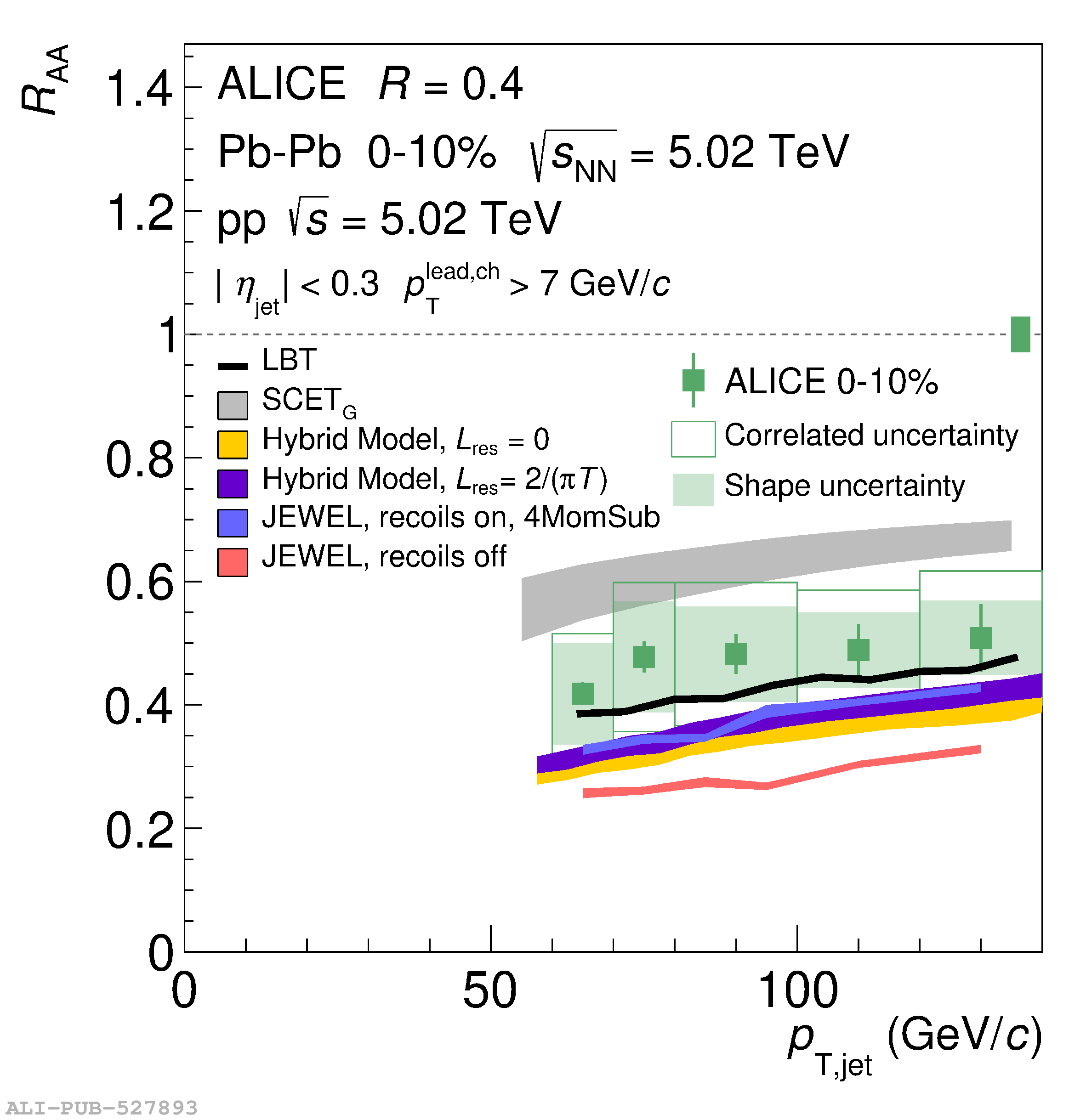# Figure 7

 Jet \Raa{} at $\sqrts=5.02$ TeV for $R=0.2$ (left) and $R=0.4$ (right) compared to LBT, SCET$_{G}$, Hybrid model, and JEWEL predictions. The combined \Taa{} uncertainty and \pp{} luminosity uncertainty of 2.8\% is illustrated as a band on the dashed line at $\Raa = 1$ Systematic uncertainties are only included for the SCET$_{G}$ and Hybrid model predictions; see text for details.